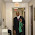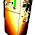### Simple Regime Change Detection with t-test

It is always fun to find trend in time series data. But what about the scenarios where the trend in the time series changes. Detecting the point of this trend change can be quite beneficial. For example, if you can immediately detect the change in revenue regime of a company it can be very valuable to that company. Or if you can detect the point that the temperature of a device starts increasing, you can possibly prevent a catastrophe! :]

In this post I aim to touch the surface for what is know as "regime change detection" or "trend change detecting" in the literature. I use a simple t-test and a rolling window approach to detect a regime change. This simple approach falls into general category of "sequential statistical tests" for regime change detection. It is also an online algorithm meaning that it can readily apply to new measurement and decide whether the same trend exist in new measurement or not.

As an example and to have a semi-practical dataset, I use hourly measurement of average duration (in second) that a visitor spends on my blog. The data belongs to a three month period. For the purpose of this post the dataset has been cleaned up, slightly modified and more importantly seasonality has been removed. In summary, we are only focusing on the trend of session duration and the goal is to detect when this trend has changed.

Let start by visualizing the data:

Clearly somewhere around 1500 hour, the average time spent on my blog is saturated. I believe the root cause is as follows: in the first two months I put some effort into spreading out my website by either asking people to go and visit it or by sharing it in different social medias. I guess after some point the blog has found its audience and my naive primitive advertising lost its effect. This is the point that the growth trend has stopped (I hope it starts growing again :] ).

### In simple words:

Let first describe the algorithm in simple words. We start by taking an initial window of data and assuming that the trend does not change in this initial window. This is the most tricky part. On one hand the size of the window needs to be large enough to establish a good model and on the other hand it should be small enough to not cover any trend change. We fit a line to this window of data.  Then we pick some points after this window and call them test points. Using t-test we see if test points trend is statistically different from the trend of the points in the window. If that hyposis is rejected we add the test points to the current window and continue with a new set of test points. We continue that till we either find a point that the hypothesis is accepted or we reach end of dataset.

### Detection:

```currentWindowSize <- 20
len <- length(df\$visit)
```
As described in simple words, the following part is iterative. I removed the loop so that I can put some comments after each part. You can find the full code in my Github.

Fit a line to current window.
```x0 <- df\$time[ 1: currentWindowSize]
y0 <- df\$visit[ 1: currentWindowSize]
c0 <- lm(y0~x0)
```
Although the modeling here is linear between x0 and y0 but it can simply be extended to nonlinear functions in x0. Pick a few data points as the test data and fit a line to the test set.
```x1 <- df\$time[ (currentWindowSize + 1): min(currentWindowSize + incrementalWindowSize, len)]
y1 <- df\$visit[ (currentWindowSize + 1): min(currentWindowSize + incrementalWindowSize, len)]
c <- lm(y1~x1)
```
Check if similarity between the trend of two lines is statistically significant. In other words we want to test the null hypothesis that the slope in c0 is equal to test slop in c:
```n = length(x1)
SSR <- sum(c\$residuals^2)
SSRx <- sum((x1-mean(x1))^2)
beta0 <- c0\$coefficients
beta <- c\$coefficients
tscore <- (beta-beta0)*sqrt(n-2)/sqrt(SSR/SSRx)
```
tscore has a student-t distribution with n-2 degree of freedom. Hence we reject the null hypothesis if:

where tau is usually set to .05 or 0.01. In simple words if the above inequality is not satisfied the two windows have same trend. If that is the case merge the test points to current window
```currentWindowSize <- currentWindowSize + incrementalWindowSize
```
If the above inequality is true, then there is a trend change in the test window.  The following plot shows the P(tscore)Red line shows the log10(p-value=0.001). p-value = 0.001 is very conservative.
So the above algorithm detect that around 1460 hour there is a change in the regime which seems good enough.

### Further Testing

I created 9 other artificial datasets to test the above approach. The error function is calculated as follows:
```residualChangeHour = actualChangeHour - calculatedChangeHour
```
The below table shows the error for each dataset. You can download the dataset and give it a try.

File name Actual Change Hour Calculated Change Hour Residual Change Hour
webvisit_0.csv -- 1460 --
webvisit_1.csv 673 680 -7
webvisit_2.csv 917 920 -3
webvisit_3.csv 1067 1080 -13
webvisit_4.csv 1023 1040 -17
webvisit_5.csv 808 820 -12
webvisit_6.csv 812 820 -8
webvisit_7.csv 1031 1040 -9
webvisit_8.csv 875 880 -5
webvisit_9.csv 523 540 -17

1.Hi Hamed, I have stock index data. How do I detect regimes in that data? Thanks.

1.Hi, It depends on the time resolution that you are interested in for stock data. If you would like to do regime detection in daily or monthly bases you can probably use the same method. However if you would like to do this in lower time resolution it is a bit tricky due to couple of reasons:
1- the underlying assumption here is that the noise is Gaussian which might no completely true for stock data
2- Each regime is length is long enough to average out noise. Stock data is highly dynamic

2.Hi Hamed,

I just wanted to check I was finding the p-value properly from this, using your variable names and the equation you have inserted, I understand you mean:
pvalue = pt(tscore, df=n-2)

However, elsewhere on the internet (http://www.cyclismo.org/tutorial/R/pValues.html) I have seen
2*pt(-abs(tscore), df=n-1).

Many thanks,
Liz

3.## Favorite Quotes

"I have never thought of writing for reputation and honor. What I have in my heart must out; that is the reason why I compose." --Beethoven

"All models are wrong, but some are useful." --George Box